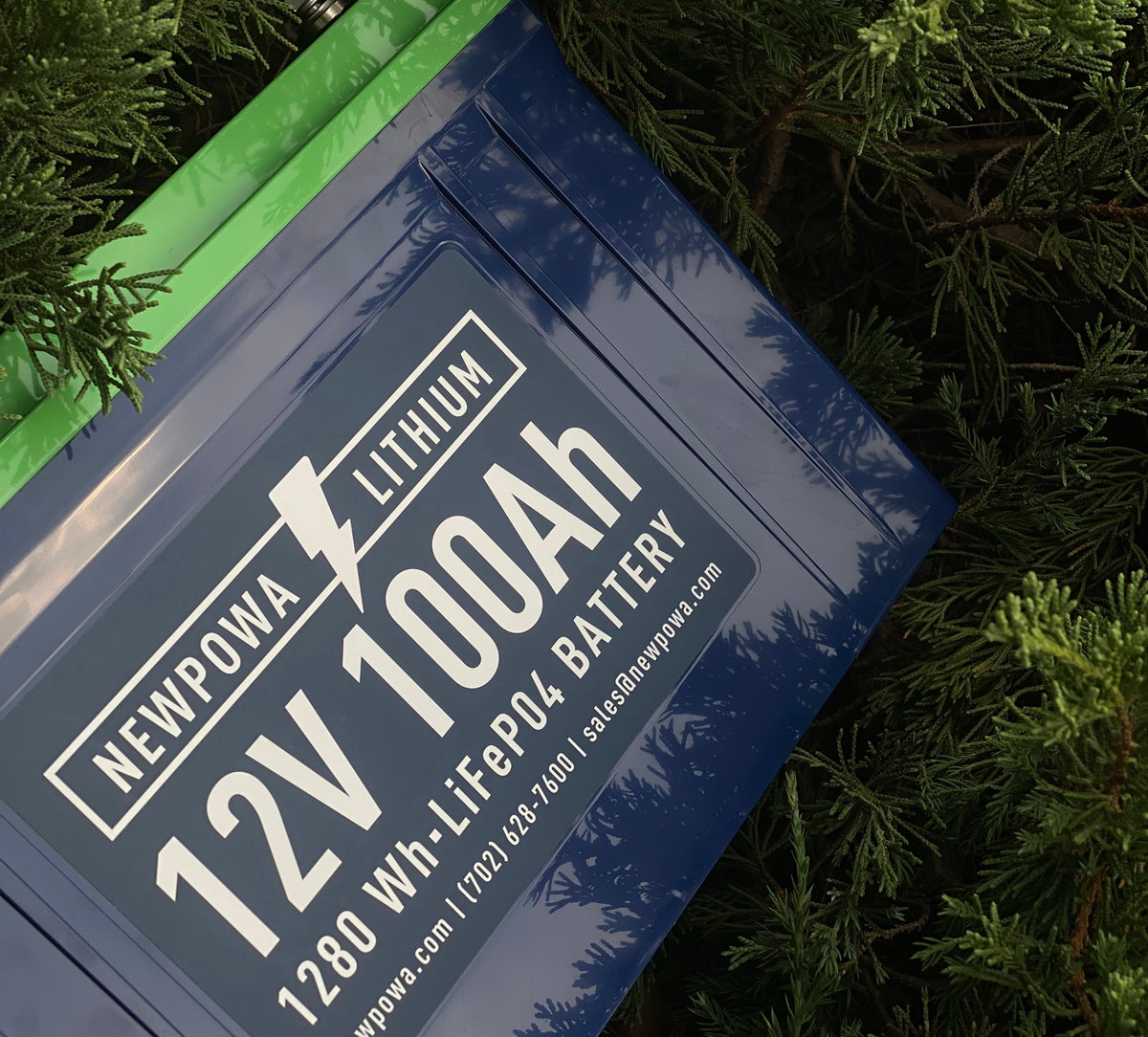# How many batteries do I need for a 3kW solar system?

When considering installing a solar system, one of the most important decisions to make is the size of the system, and specifically, the number of batteries needed. This decision can impact the system's performance, longevity, and overall cost.

For a 3kW solar system, the number of batteries required depends on several factors, including the battery capacity, the solar panel output, and the expected daily power usage.

First, let's define what a 3kW solar system is. A 3kW solar system typically consists of 10 solar panels, each with an output of 300 watts. This system can generate an average of 12-15 kilowatt-hours (kWh) of electricity per day, depending on factors such as location, weather conditions, and the orientation of the solar panels.

To determine the number of batteries required for a 3kW solar system, we need to consider the battery capacity. A common battery capacity used in solar systems is 100 Ah (ampere-hour). To calculate the total battery capacity needed, we need to multiply the daily power usage by the number of days of backup power required.

For example, if the daily power usage is 10kWh and backup power is required for two days, then the total battery capacity required is 20kWh. To convert this to Ah, we can use the formula:

20,000 Wh / 24 volts = 833.33 Ah

This means we would need eight to nine batteries with a capacity of 100Ah each to power a 3kW solar system for two days. However, it's important to note that this is just an estimate, and the actual number of batteries required may vary depending on several factors such as the battery technology, efficiency, and discharge depth.

Another factor to consider when determining the number of batteries needed for a solar system is the charge controller. A charge controller is an important component that regulates the amount of power flowing between the solar panels and the batteries. An efficient charge controller can ensure that the batteries are charged quickly and efficiently, thereby reducing the number of batteries required for a solar system.

In conclusion, the number of batteries required for a 3kW solar system depends on several factors, including the battery capacity, the daily power usage, and the expected backup time. As a general rule of thumb, a 3kW solar system will require around eight to nine 100Ah batteries for backup power of two days. However, it's important to consult with a professional solar installer to determine the exact number of batteries required for your specific solar system needs.